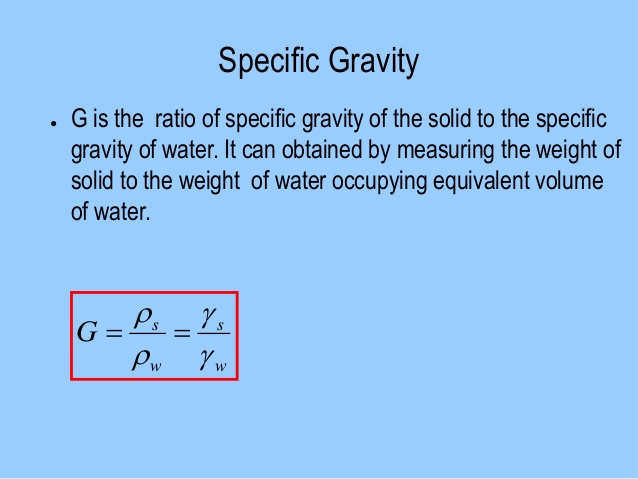Feb 26, 2016

Feb 26, 2016

Feb 26, 2016

# To Determine the Specific Gravity of SoilASTM Designation: C128

## Apparatus

Sieve #4, balance, electric oven, pycnometer.

## Theory

Specific gravity is defined as the ratio of the weight of given volume of material to the weight of an equal volume of water.

G = density of soil/density of equal volume of water

G = mass of dry soil/mass of and equal volume of water.

## Procedure

• Take at least 25g of soil which has been passed through sieve#4 and place it in an oven at fixed temperature of 105-110 °C for 24 hours to dry it completely.
• Clean and dry the pycnometer thoroughly and find its mass (M1).
• Find the mass (M2) of pycnometer by placing dried soil in it.
• Add sufficient quantity of water to fill the pycnometer up to the given mark and then find mass of the pycnometer ( m3) and its content.
• Empty the pycnometer then fill it with water up to the same level. Now find the mass (M4) of the pycnometer having water in it.
• Determine the specific gravity of the given soil sample.

## Precautions

• The graduated cylinder used should be cleaned.
• Dry the coarse aggregate so that it does not absorb moisture otherwise it will not give the desired results.
• All the readings of mass should be noted carefully.

## Practical applications

• The value of specific gravity helps us to some extent in identification and classification of soil.
• It gives the idea about the suitability of a given soil as a construction material.
• It is utilized in calculating voids ratio, porosity, and degree of saturation if the density or unit weight and water content are known.